## The GLM model

Fitting a GLM to expression data

It is easiest to understand how the GLM model works through an example. Imagine an experiment looking at the effect of two drug treatments while controlling for gender:

• Test differential expression due to Treatment with three groups: drugA, drugB, placebo
• While controlling for Gender with groups: Male, Female

In an abuse of mathematical notation, the underlying GLM for each gene looks like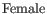(31.1)

where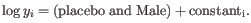is the expression level for the gene in sample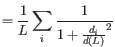; the combined term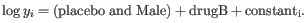describes an arbitrarily chosen baseline expression level (of males being given a placebo); and the other terms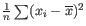,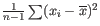and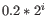are numbers describing the effect of each group with respect to this baseline. The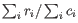accounts for differences in the library size between samples. For example, if a subject is male and given a placebo we predict the expression level to be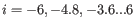If instead he had been given drug B, we would predict the expression levelto be augmented with thecoefficient, resulting in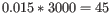We assume that the expression levelsfollow a Negative Binomial distribution. This distribution has a free parameter, the dispersion. The greater the dispersion, the greater the variation in expression levels for a gene.

The most likely values of the dispersion and coefficients,,and, are determined simultaneously by fitting the GLM to the data. To see why this simultaneous fitting is necessary, imagine an experiment where we observe counts {15,10,4} for Males and {30,20,8} for Females. The most natural fit is for the coefficientto have a two-fold change and for the dispersion to be small, but an alternative fit has no fold change and a larger dispersion. Under this second fit the variation in the counts is greater, and it is just by chance that all three Female values are larger than all three Male values.

Refining the estimate of dispersion

Much research has gone into refining the dispersion estimates of GLM fits. One important observation is that the GLM dispersion for a gene is often too low, because it is a sample dispersion rather than a population dispersion. We correct for this using the Cox-Reid adjusted likelihood, as in the multi-factorial edgeR method [Robinson et al., 2010]. To understand the purpose of the correction, it may help to consider the analogous situation of calculation of the variance of normally distributed measurements. One approach would be to calculate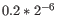, but this is the sample variance and often too low. A commonly used correction for the population variance is:.

A second observation that can be used to improve the dispersion estimate, is that genes with the same average expression often have similar dispersions. To make use of this observation, we follow [Robinson et al., 2010] in estimating gene-wise dispersions from a linear combination of the likelihood for the gene of interest and neighboring genes with similar average expression levels. The weighting in this combination depends on the number of samples in an experiment, such that the neighbors have most weight when there are no replicates, and little effect when the number of replicates is high.

When estimating dispersion, we use the following strategy:

1. We sort the genes from lowest to highest average logCPM (CPM is defined also by the edgeR authors). For each gene, we calculate its log-likelihood at a grid of known dispersions. The known dispersions are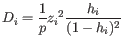, where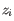such that there are 11 values of i in total. You can imagine the results of this as being stored in a table with one column for each gene and one row for each dispersion, with neighboring columns having similar average logCPM (because of the sorting in the previous step).

2. We now calculate a weighted log-likelihood for each gene at these same known dispersions. This is the original log-likelihood for that gene at that dispersion plus a "weight factor" multiplied by the average log-likelihood for genes in a window of similar average logCPM. The window includes the 1.5% of genes to the left and to the right of the gene we are looking at. For example, if we had 3000 genes, and were calculating values for gene 500, then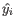, so we would average the values for genes 455 to 545. The "weight factor" = 20 / (numberOfSamples - number of parameters in the factor being tested). This means that the greater the number of samples, the lower the weight, and the fewer free parameters to fit, the lower the weight.

3. We fit an FMM spline for each gene to its 11 weighted log-likelihoods. Our implementation of the FMM spline is translated from the Fortran code found here http://www.netlib.org/fmm/spline.f. This spline allows us to estimate the weighted log-likelihood at any dispersion within the grid i.e., from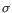to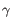4. We find the dispersion on the spline that maximizes the log-likelihood. This is the dispersion we use for that gene.

#### Downweighting outliers

It is often difficult to detect outliers. Therefore, instead of removing putative outliers completely from the GLM fit, we give them "observation weights" that are smaller than 1. These weights reduce the effect of the outliers on the GLM fit.

Outliers can have two undesirable effects. First, genes with outliers are more likely to be called as differentially expressed because a sample with anomalously high expression is hard to reconcile with no fold change. Second, the presence of outliers can prevent differentially expressed genes from being detected. This is because, when a gene has an anomalously high expression, the dispersion for that gene will typically be overestimated. This will in turn cause the dispersion of genes with similar expression to be overestimated, such that they are less likely to be called as differentially expressed.

When downweighting outliers, the above dispersion estimation procedure is repeated 5 more times, and per-gene weights are estimated for the samples at each iteration. These weights are not related to the weights in the weighted log-likelihood of the dispersion estimation procedure. The following iterative procedure is performed to estimate the observation weights. For each gene, one iteration proceeds as follows:

1. The Cook's distance is calculated for each sample. The Cook's distance for sampleis: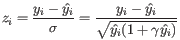where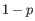is the number of parameters in the fit,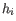is the Pearson residual for sample, which is a measure of how well the fitted model predicts the expression of sample, and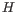is the leverage of sampleon the fit, which is a measure of how much sampleaffects the fitted parameters.

Outliers are expected to have large Cook's distances because they are unlikely to be fit well, leading to large, and they are likely to distort the fit disproportionately to the other points, leading to large.

In more detail, the Pearson residual,is the difference between the measured expressionand the modeled expression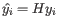, divided by the standard deviation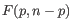of the negative binomial distribution with dispersion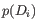that has been fitted to the gene: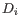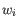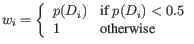The leverageis the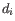entry on the diagonal of the projection matrix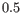which relates measured expressions to modeled expressions: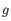.

2. Cook's distances are assumed to follow an F distribution,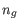where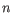is the number of samples. For each sample we use this distribution to calculate the probability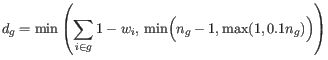of obtaining an equally or more extreme Cook's distance than the observed one. The total downweighting for the gene is: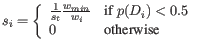where: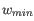The interpretation of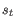is that the algorithm is allowed to disregard at most one sample or up to 10% of the data - whichever is larger. In practice, the weights are spread across all samples: one sample may be more heavily downweighted than another, but it is unusual for a sample to be completely ignored. The probability cut-off of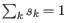is set empirically from experiments on real data.

3. The observation weight for sampleis: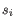The scaling of the observation weights by the most extremespeeds up the detection of outliers. Typically one outlier in a condition will affect the fit of all the other replicates of the condition such that they also have large Cook's distances and appear to be outliers. If the real outlier has a 10x smallerthan the replicate, then the scaling will start by giving the real outlier a 10x smaller observation weight.
4. The GLM is re-fit, using these weights.

The above steps are repeated until no weight changes by more than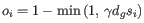, or a weight that was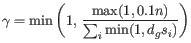becomes 1 in the following round, or a weight that decreased by more thanin the previous round increases by more thanin the next round. These stopping criteria are needed because the weights do not converge: when a weight becomes sufficiently small, the leverage component of the Cook's distance tends to zero and so the Cook's distance also becomes zero and the weight in the next iteration will be 1.

Once the observation weights have been determined, the re-fitted GLM has a different likelihood than before. This is partly because the weighting is applied directly to the likelihood, and partly because the weighting affects the fitted coefficients.

The use of observation weights in the GLM is based on [Zhou et al., 2014]. The use of Cook's distance is motivated by DESeq2, which ignores genes with extreme Cook's distances when the number of replicates is small, rather than downweighting them. The form of the weights is novel to CLC Genomics Workbench.

Statistical testing

The final GLM fit and dispersion estimate allows us to calculate the total likelihood of the model given the data, and the uncertainty on each fitted coefficient. The two statistical tests each make use of one of these values.

Wald test
Tests whether a given coefficient is non-zero. This test is used in the All group pairs and Against control group comparisons. For example, to test whether there is a difference between subjects treated with a placebo, and those treated with drugB, we would use the Wald test to determine if thecoefficient is non-zero.
Likelihood Ratio test
Fits two GLMs, one with the given coefficients and one without. The more important the coefficients are, the greater the ratio of the likelihoods of the two models. The likelihood of both models is computed using the dispersion estimate and observation weights of the larger model. This test is used in the Across groups (ANOVA-like) comparison. If we wanted to test whether either drug had an effect, we would compare the likelihoods of the GLM described in equation 31.1 with those in the reduced GLM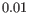.